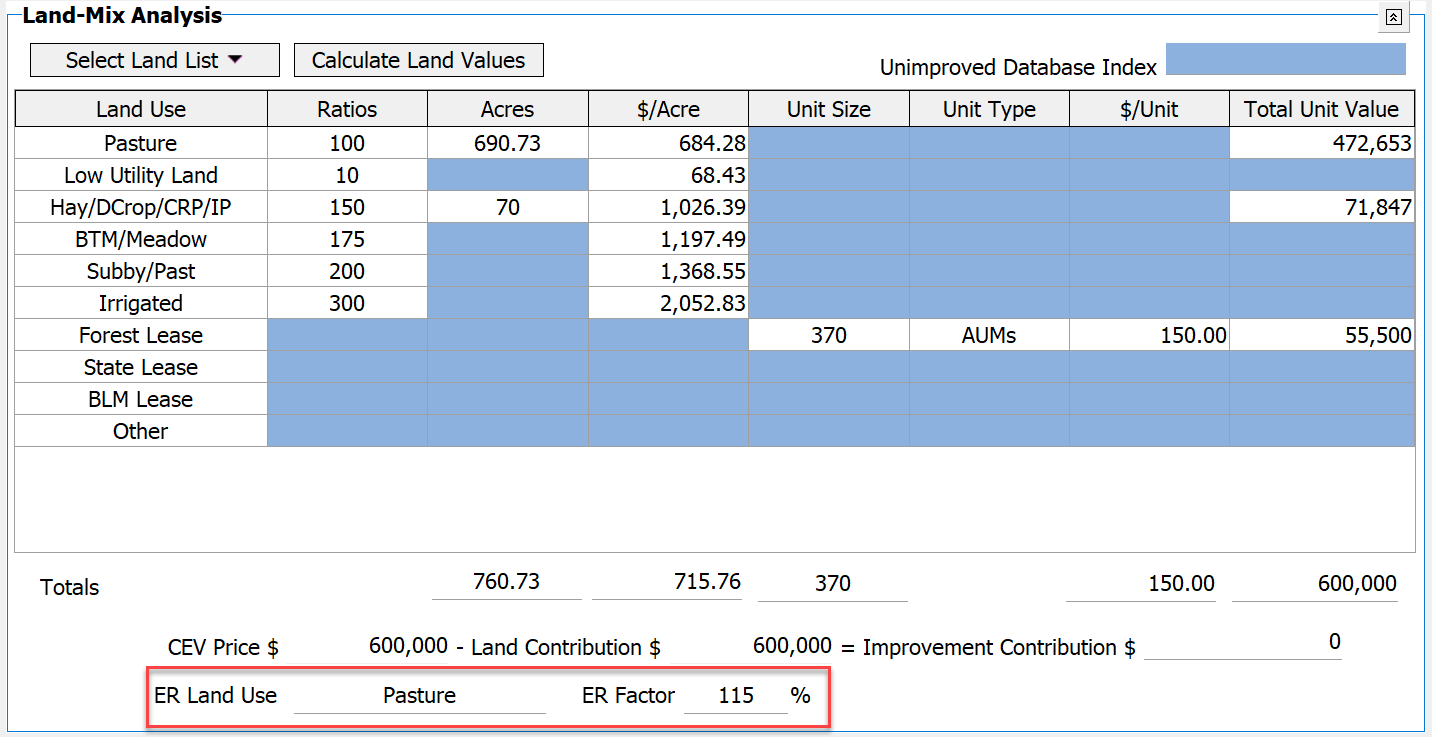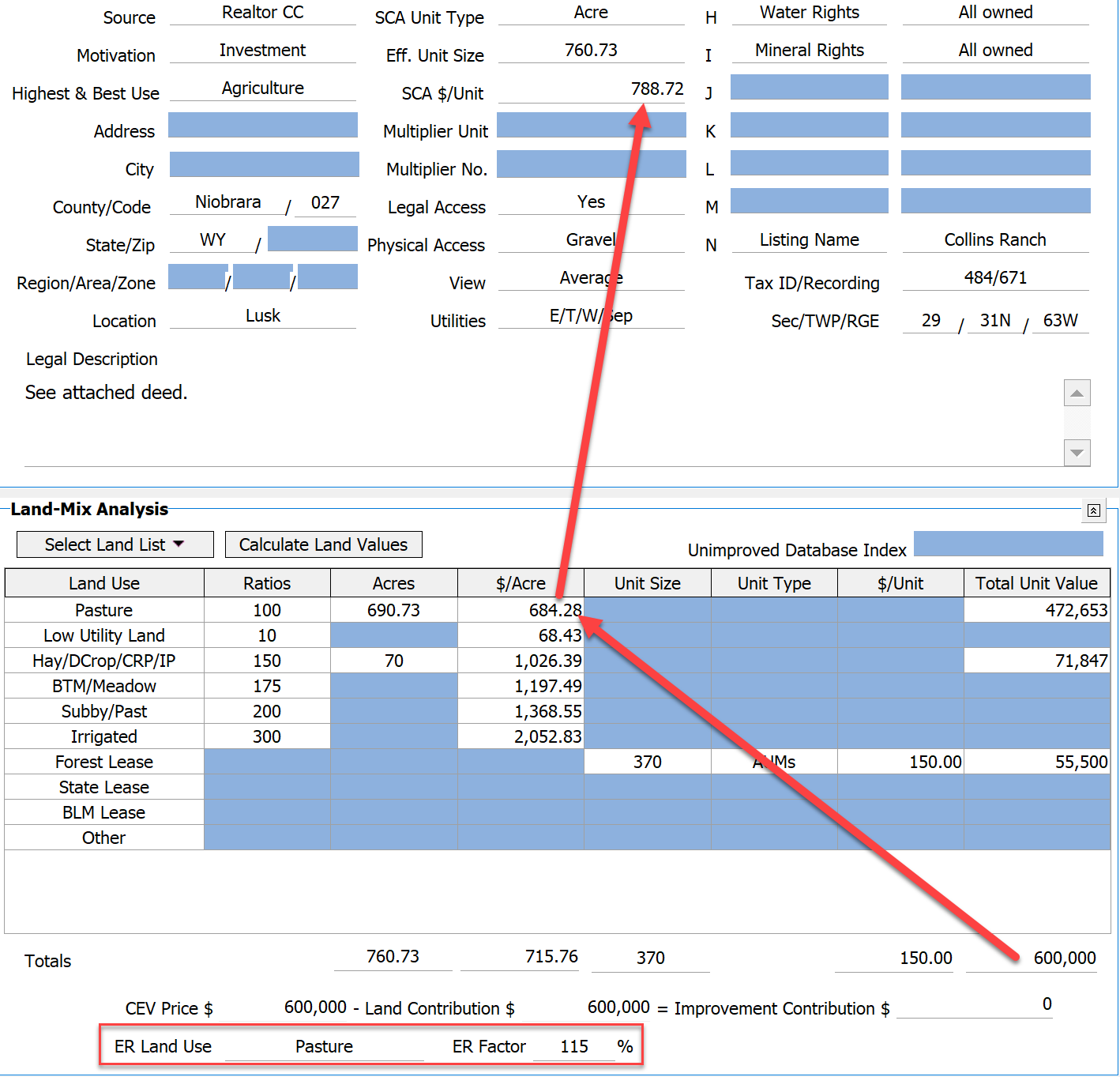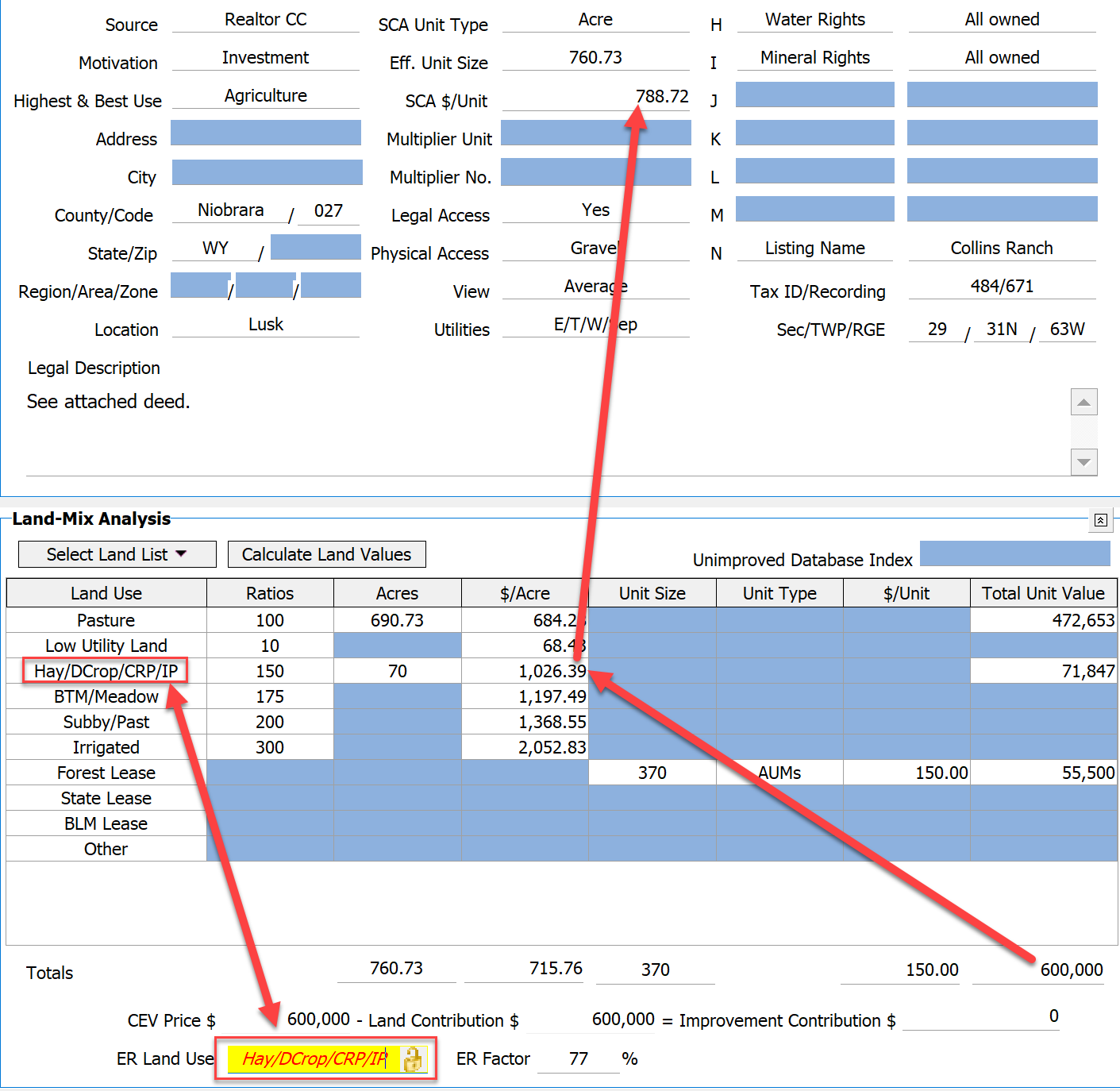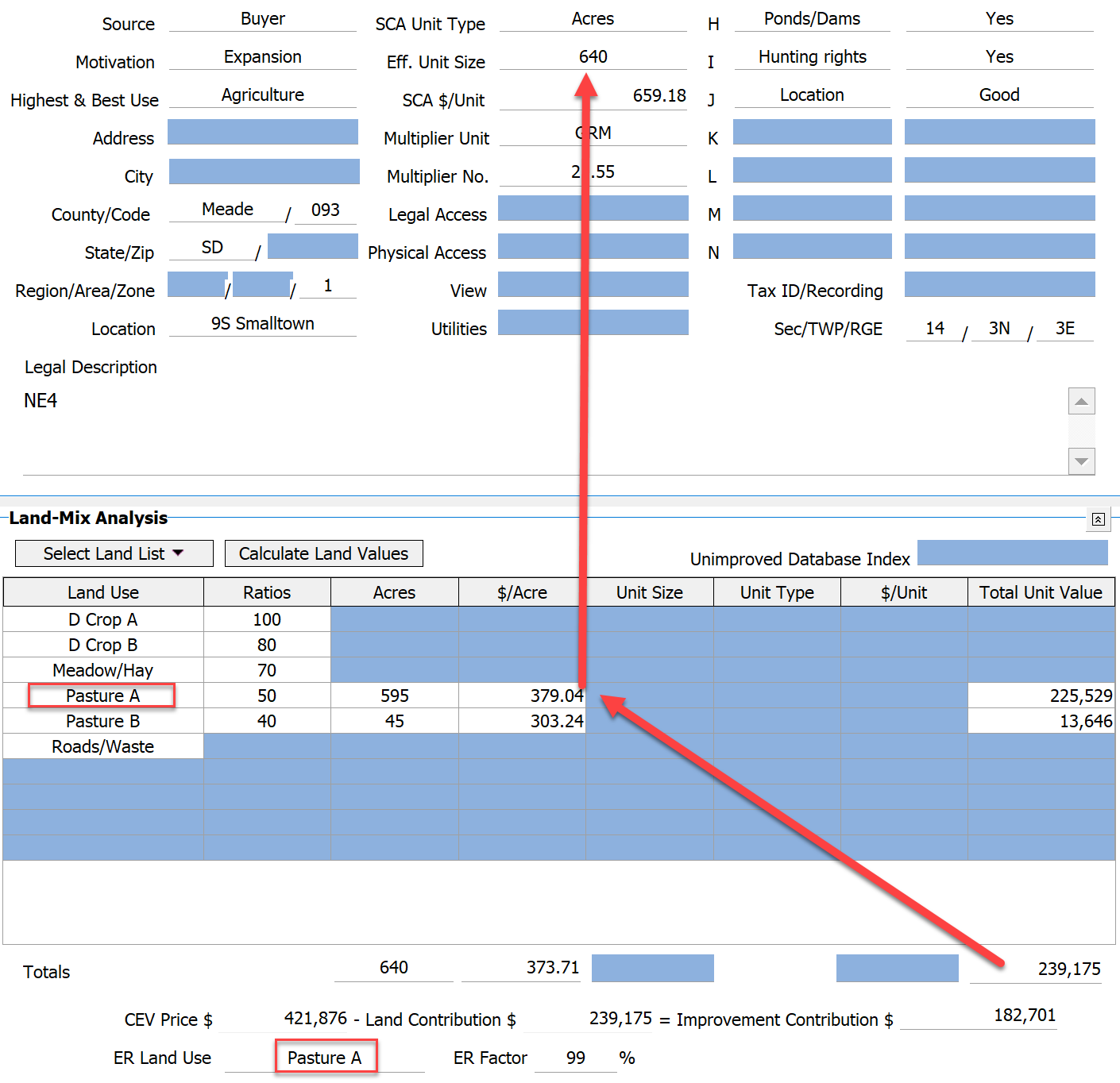The new ASFMRA appraisal textbook uses the “ER Factor” for making land adjustments. This is an “Equivalency Ratio” or “Equivalency Rating” depending on preference.  ER Ratio is defined as a mathematic expression (percentage) of each property’s land mixture or composition.

AgWare added 2 new fields in DataLog, located at the bottom of the Land Mix Analysis Section:

• “ER Land Use” and
• “ER Factor”  (additional explanation in article titled “3 Ways to Calculate the ER”)The formula to calculate the ER Factor is (Total Land Value / \$/Acre of the “top dollar” at 100% or Pasture in this example) / Eff. Unit Size * 100

So assuming an Effective Unit Size of 760.73,
(600,000 / 684.28) /760.73 * 100 = 115% (rounded)

Normally, ER Land Use is the “Land Use” Name of the first row in the land table that has a “Dollar Per Acre Value” displayed (\$684.28/acre in this example).

• NOTE:  If the very first row (Pasture) had a \$/Acre value of zero, DataLog would apply the “ER Land Use” shown for the second row (“Low Utility Land” or \$64.43/acre in this example).  Thus, it is important for the user to select sales with the predominant “Land Use” for best results.

The user can unlock the ER Land Use cell and select a different Land Use, the math is automatically triggered to reflect the ER Factor for that land use.

The ER Factor can be greater or less than 100%.  If the Effective Unit Size is equal to total acres, and only one land type and dollar per acre displayed, then the ER is 100%. If there are additional acres at values at more than the baseline (ER Land Use), then the value will be greater than 100% (like the prior example). If there are acres valued at less then the ER will be less than 100%.

The calculation must be tiered. The Land use is set first, then the value based on that row. If the user “overrides” the land use name and types in a value, the ER Factor is calculated based on that new or “over-ridden” value.

 By default the first land row with a \$/Acre is usedFirst land row with \$/Acre is D Crop AExample unlocking the cell and manually naming the ER Land Use: Unlocking and renaming the field to another valid land row uses that \$/AcreAnother Example this time showing that the first land row with a \$/Acre is used: